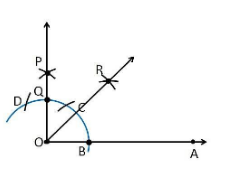Guru

# Construct an angle of 45° at the initial point of a given ray and justify the construction. Q.2

• 0

Please guide me the best way for solving the question of class 9th math of Constructions chapter of exercise 11.1of math of question no.2  What is the best way for solving this question, please guide me Construct an angle of 45° at the initial point of a given ray and justify the construction.

Share

1. Construction Procedure:

1. Draw a ray OA

2. Take O as a centre with any radius, draw an arc DCB is that cuts OA at B.

3. With B as a centre with the same radius, mark a point C on the arc DCB.

4. With C as a centre and the same radius, mark a point D on the arc DCB.

5. Take C and D as centre, draw two arcs which intersect each other with the same radius at P.

6. Finally, the ray OP is joined which makes an angle 90° with OP is formed.

7. Take B and Q as centre draw the perpendicular bisector which intersects at the point R

8. Draw a line that joins the point O and R

9. So, the angle formed ∠ROA = 45°Justification

From the construction,

∠POA = 90°

From the perpendicular bisector from the point B and Q, which divides the ∠POA into two halves. So it becomes

∠ROA = ½ ∠POA

∠ROA = (½)×90° = 45°

Hence, justified

• 0